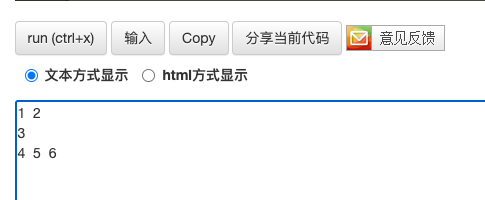• 初学c++，定义了一个int arr; 我想求arr 的实际长度是多少，没找到方法，... // 模拟数据，int数组arr 实际长度 为 3 int len = end(arr)-begin(arr); int len2 = sizeof(arr) / sizeof(arr); cout <&.

初学c++，定义了一个int arr;  我想求arr 的实际长度是多少，没找到方法，自己写了一个方法，不知道是否可行。

int arr;
arr = 1;
arr = 2;
arr = 3;
// 模拟数据，int数组arr 实际长度 为 3

int len = end(arr)-begin(arr);
int len2 =  sizeof(arr) / sizeof(arr);
cout <<"-----len-------"<< len << endl;
cout <<"-----len2-------"<< len2 << endl;
// 以上求长度方法打印的 int数组arr 为 5

// 求int 数组arr 的实际长度
int size = 0;
for(int i = 0; i < 5;i++) {
if(arr[i]) {
cout <<"-----arr[i]-------"<< arr[i] << endl;
size ++;
}
}
cout <<"-----total size-------"<< size << endl; // 3

//---------------------------------------------------
// 求 vector 数组data长度
vector<int> data = {1, 2, 3, 4};

int datalen = end(data)-begin(data);
int datalen2 =  data.size();
cout <<"-----datalen-------"<< datalen << endl;
cout <<"-----datalen2-------"<< datalen2 << endl;

打印如下：展开全文c++
• //以初始化列表中的値作为元素初始值，生成有6个数的初始vector数组 vector<int> array = {1,2,3,4,5,6}; vector<int> array{1,2,3,4,5,6}; //第三种初始化，使用初始元素个数以及指定元素

一. 一维数组
1. 数组初始化

//第一种初始化，就单纯的建立一个可变数组array，因为还没有存放元素，未分配空间
vector<int> array;

//第二种初始化，是使用初始化列表来指定初始值以及元素个数
//以初始化列表中的値作为元素初始值，生成有6个数的初始vector数组
vector<int> array = {1,2,3,4,5,6};
vector<int> array{1,2,3,4,5,6};

//第三种初始化，使用初始元素个数以及指定元素值来生成初始vector数组
//生成一个具有numbers个元素的vector数组，且数组中所有元素初始化为value
vector<int> array(numbers, value);
vector<int> array(numbers);//此时，默认value为0
//第四种初始化，使用复制另一个数组的方式来初始化一个新数组,
//表示复制前n个元素
vector<int> newArray(array.begin(), array.begin() + n);//n = 3: newArray{1,2,3}

2. 数组的访问操作

//	 第一种：通过下标访问，与普通数组一样
array;	// 1
array;	// 2
array;	// 3
array;	// 4
array;	// 5
array;	// 6
//	第二种：通过迭代器访问
vector<int>::iterator it;	// 迭代器it的定义
it = array.begin();
// 使用auto定义迭代器it也可
auto it = array.begin();
for(int i = 0 ; i < 6 ; i ++) {
cout<<*(it + i)<<" ";
}

3. 数组的遍历操作（auto定义）

//C++11标准，auto关键字遍历
for(auto iter = array.begin(); iter != array.end(); iter ++){
cout << *iter <<" ";
}
// 也可以将下列中的auto换成定义的int型
for(auto iter : array)
{
cout<<iter<<" ";
}

4. 数组的其他操作

//添加元素
array.push_back(7);//将元素7加入数组末尾
//删除元素
//	1.删除数组末尾的元素
array.pop_back();
//	2.通过erase(iter)，单个删除迭代器为iter处的元素
auto iter = array.begin();
array.erase(iter);//删除array
//	3.通过erase(first,last)，批量删除一个区间[first, last)内的所有元素
array.erase(array.begin() + 1, array.begin() + 3);//删除array,array
//插入元素
//	insert(iter, x)用来向vector的任意迭代器iter处插入一个元素x
auto iter = array.begin() + 1;
array.insert(iter, 9);//将元素9插入array的位置
//清空所有元素
array.clear();
//获取数组长度
array.size();
//判空
array.empty();

二. 二维数组
1. 数组初始化
（1）定义的同时初始化元素值

vector<int> cov(3);//定义3个值为0的数组，具体参见上述一维数组的初始化
int row = 3;
vector<vector<int>> arr(row, cov);//row为行数，cov为列数上的数组，初始化成一个3*3的0元素矩阵
//或者换成另一种写法
int row = 3;
vector<vector<int>> vec(row, vector<int> (col,1));//col代表元素个数，也就是列数，1为元素默认值，若只有col，则默认值为0

（2）先通过resize函数初始化容器大小，然后赋值
函数原型：
void resize (size_type n);
void resize (size_type n, const value_type& val);
第一个参数是将容器的元素变成n个，第二个参数是将这n个元素变成val（没有就是默认值）
注意：resize不适用于array
如果当前大小大于所要求的大小，容器后部的元素会被删除；如果当前大小小于新大小，会将新元素添加到容器后部。

vector<vector<int>> arr;
int row = 3;
int col = 3;
arr.resize(row);//定义行数大小
for(int i = 0; i < arr.size(); i++) {
arr[i].resize(col);//定义每一列的大小
}
for(int i = 0; i < row; i++) {
for (int j = 0; j < col; j++) {
arr[i][j] = 1;
}
}
//	或者另一种写法
vector<vector<int>> arr;
int row = 3;
int col = 3;
arr.resize(row, vector<int>(cov, 1));
for(int i = 0; i < row; i++) {
for (int j = 0; j < col; j++) {
arr[i][j] = 1;
}
}

2. 数组的遍历操作
（1）迭代器遍历

for(auto iter = arr.begin(); iter != arr.end(); iter++)
{
auto vec_tmp = *iter;
for(auto it = vec_tmp.begin(); it != vec_tmp.end(); it++)
cout << *it << " ";
cout << endl;
}

（2）下标遍历

for (i = 0; i < arr.size(); i++)
{
for(j = 0; j < arr.size(); j++)
cout << arr[i][j] << " ";
cout << endl;
}

3. 数组的其他操作

//	添加元素
arr[i].push_back(1);
//	如下类似

展开全文c++ 开发语言
• 一.概念 vector<type> 容器是包含 type 类型元素的序列容器。...二.vector数组的创建 vector<type> name; type:指数组类型，例如int,float,double,string等等。 name: 创建的数组的名称，想取什么取

一.概念

vector<type> 容器是包含 type 类型元素的序列容器。
vector<type> 容器的大小(长度)可以自动增长，从而可以包含任意数量的元素，只要元素个数超出 vector 当前容量，就会自动分配更多的空间。
但是只能在容器尾部高效地删除或添加元素。

二.vector数组的创建

vector<type> name;
type:指数组类型，例如int,float,double,string等等。
name: 创建的数组的名称，想取什么取什么。

三.vector数组的初始化

//第一种初始化，就单纯的建立一个可变数组array，因为还没有存放元素，未分配空间
vector<int> array;

//第二种初始化，是使用初始化列表来指定初始值以及元素个数
//以初始化列表中的値作为元素初始值，生成有6个数的初始vector数组
vector<int> array = {1,2,3,4,5,6}
vector<int> array{1,2,3,4,5,6}

//第三种初始化，使用初始元素个数以及指定元素值来生成初始vector数组
//生成一个具有numbers个元素的vector数组，且数组中所有元素初始化为value
vector<int> array(numbers, value);
vector<int> array(numbers);//此时，默认value为0

展开全文c++
• #include<iostream> #include<vector> using namespace std; //目标就是完成二维vector的所有... //二维数组vector 定义 // 构造一维的vector vector<int> vec1; vec1.push_back(1);.
#include<iostream>
#include<vector>

using namespace std;

//目标就是完成二维vector的所有操作
int main(void)
{

vector<vector<int>> vec2; //二维数组的 vector 定义

// 构造一维的vector
vector<int> vec1;

vec1.push_back(1);
vec1.push_back(2);
// 将vec1插入到vec2中
vec2.push_back(vec1);

vec1.clear();
vec1.push_back(3);
vec2.push_back(vec1);

vec1.clear();
vec1.push_back(4);
vec1.push_back(5);
vec1.push_back(6);
vec2.push_back(vec1);

//打印“二维数组”，本处演示先按列打印
for(int i = 0; i < vec2.size(); ++i){
for(int j = 0 ; j< vec2[i].size(); ++j){
cout << vec2[i][j] << "  ";
}
cout << endl ;
}
}展开全文c++
• 任意一维数组的输入输出 那么,我们可以借鉴之前的思路,使用两个scanf("%d",&temp)来嵌套输入,这样一来思路就很明确了. #include<iostream> #include<vector> #include<cstdio> using ...c++ 开发语言 后端
• 主要是记住一维和二维数组的定义以及区别，它们初始化，和添加删除元素是有点不太一样的。 一维数组 vector<int> arr; arr.size(); arr.begin(); arr.insert(); arr.push_back(); arr.end(); 二维数组 ...
• VECTOR数组

2021-02-14 20:12:51
Vector是一个封装了动态大小数组的顺序容器（Sequence Container）。跟任意其它类型容器一样，它能够存放各种类型的对象。可以简单的认为，vector是一个能够存放任意类型的动态数组。 可以这样说，vector就是一个...数据结构 c++ 算法 数组 stl
• 一维数组 二维数组 vector<int> res(10); vector<vector<int>> res(10,vector<int>(10,INT32_MAX))c++
• vector数组对象元素访问与普通数组相同： 数组对象名[下标表达式] 注意，vector数组对象不是数组，而是封装了数组的对象，vector数组名字表示的就是一个数组对象，而非数组的首地址。 vector定义数组对象成员函数...
• 数组一维数组初始化方式：1. int []arr=new int; 和int arr[] = new int;效果是一样的，不过开发中推荐前者。2. int[]arr = new int[]{3,5,1,7};或者简化为int []arr = {3,5,1,7};二维数组定义方式//直接为每...
• 数组对象名(数组长度); 尖括号中的类型名表示数组元素的类型。数组长度是一个表达式，表达式中可以包含变量。 #include <iostream> #include <vector> using namespace std; // 计算数组arr 中元素的...
• vector本来就是可以用来代替一维数组的，vector提供了operator[]函数，可以像数组一样的操作，而且还有边界检查，动态改变大小。这里只介绍用它来代替二维的数组，二维以上的可以依此类推。1、定义二维vectorvector ...
• 通过引用一个数组来读取起长度 (&arr)先前调其为引用，_Ty [_Size]指出起引用值的类型 template<typename _Ty, size_t _Size> size_t GetArrSize( _Ty(&arr)[_Size]) { return _Size; } 2.模板函数2...
• 去除数组中指定元素，得到新数组长度 题目：给你一个数组nums和一个值val,你需要原地移除所有数值等于va的元素,并返回移除后数组的新长 度 不要使用额外的数组空间,你必须仅使用O(1)额外空间并原地修改输入数组。 ...leetcode c++ 指针
• 删除排序数组中的重复项,返回数组长度 一、题目 给定一个排序数组，你需要在原地删除重复出现的元素，使得每个元素只出现一次，返回移除后数组的新长度。 不要使用额外的数组空间，你必须在原地修改输入数组 并在...leetcode 数据结构 c++ 指针 数组
• 2、声明 （1）类型 数组名[数组长度]…[n]; 注意： ①数组长度必须是一个大于等于0的常量 ②数组的下标从0开始访问 （2）普通数组的声明 //判断一个对象是不是常量表达式 const int max = 20; //是常量表达式 const...c++ 数据结构 数组 stl
• 题意： 给你一个整数数组 nums，请你求出乘积为正数的最长子...请你返回乘积为正数的最长子数组长度。 方法：动态规划，定义dp数组，存储前i个数字里，乘积为正数的最长子数组 class Solution { public: i..leetcode 动态规划
• 如果作者在20世纪90年代早期将数组定义为可变长度,则会产生一些不必要的后果：>数组的核心JVM定义会复杂得多,并且所有实现都将被迫在低级别提供这种复杂性;>在给定的JVM中会有一个“一刀切”的大小调整实现,这...java数组规定长度
• C++普通数组转vector

千次阅读 2020-12-21 17:13:55
std::vector，内部存储的所有元素，都是占用一片连续的内存空间。因此，比较好的做法就是直接取第一个元素的地址，得到了首地址，之后的做法与数组也是大同小异了。 举例说明。 假设函数Func需要以数组的方式传递，...vector 数组
• 相比较于vector数组的缺点前言数组的缺点数组的灵活性差数组不允许拷贝和赋值数组更容易越界获取数组长度不方便数组数组结束语 前言 数组vector一样可以存放任意对象，除了引用，即不存在引用的数组，也不...
• 1. array 定义的时候必须定义数组的元素个数;而vector 不需要；　且只能包含整型字面值常量，枚举常量或者用常量表达式初始化的整型const对象，非const变量以及需要到运行阶段才知道其值的const变量都不能用来定义...
• 接下来要给大家带来的是找出数组中出现的次数超过数组长度的一半的一个数字的java实现和思路，一起来看看吧。题目：数组当中有一个数字出现的次数超过了数组长度的一半，请摸找出这个数字。例：输入一个长度为9的...
• 在一个二维数组中（每个一维数组长度相同），每一行都按照从左到右递增的顺序排序，每一列都按照从上到下递增的顺序排序。请完成一个函数，输入这样的一个二维数组和一个整数，判断数组中是否含有该整数。 示例1 ...vector 二维数组
• 请你返回乘积为正数的最长子数组长度。 示例 1： 输入：nums = [1,-2,-3,4] 输出：4 解释：数组本身乘积就是正数，值为 24 。 示例 2： 输入：nums = [0,1,-2,-3,-4] 输出：3 解释：最长乘积为正数的子数组为 [1,-2...动态规划 leetcode 算法 数据结构
• 1.vector的定义 2. vector内的元素访问 3. vector常用函数 push_back(x) pop_back() size() clear() insert(it,x) erase(it)和erase(first,last) 4. vector常见用途 1.存储数据 2.用邻接表存储图 1....STL vector
• 1.有序数组不允许有重复元素 思路：定义2个变量i，j ，俗称快慢指针 1.初始值：慢指针i从下标为0的元素开始，快指针j.../*入参为nums有序数组，n为数组长度*/ int removeDuplications(int *nums,int n) { int i=0,j数据结构
• 给定一个数组 nums 和一个目标值 k，找到和等于 k 的最长子数组长度。如果不存在任意一个符合要求的子数组，则返回 0。 注意: nums 数组的总和是一定在 32 位有符号整数范围之内的。 示例 1: 输入: nums = [1, -1,...leetcode 算法
• int fun(vector<int> arr) { map<int, int> is_key; int len = 0;//最大长度 int tmp = 0;//当前长度 for(int i = 0; i < arr.size(); i++) { if(is_key.find(arr[i]) != is_key.end()) { i ...
• casting in stone a variable-length mechanism already adequately handled by higher-level structures (java.util.Vector back in the day, and now Java Collections, Guava etc). To sum up, arrays in the C-...
• 给定一个数组 nums 和一个目标值 k，找到和等于 k 的最长连续子数组长度。如果不存在任意一个符合要求的子数组，则返回 0。c++ leetcode 开发语言...